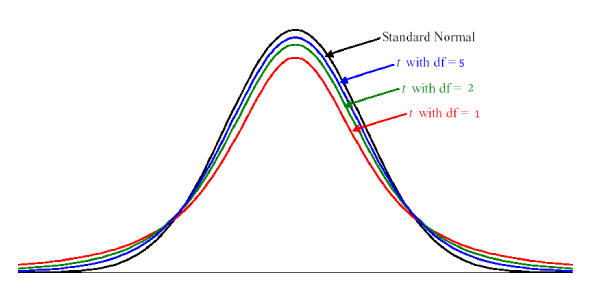# T Test MCQ Quiz With Answers

10 Questions | Attempts: 29294
ShareSettingsHow much do you know about the T-test? Think you can pass a quiz? Here, we present to you a T-Test MCQ Quiz with answers. A T-test is a very useful inferential statistic used that is to determine if there is a noticeable difference between the means of two groups. Do you think you can pass this test? Why not try now and get to know yourself? Without wasting a moment, we should directly jump into the quiz—best of luck to you before you start.

• 1.
A t-test is a significance test that assesses
• A.

The means of two independent groups

• B.

The medians of two dependent groups

• C.

The modes of two independent variables

• D.

The standard deviation of three independent variables

• 2.
To use a t-test, the dependent variable must have
• A.

Nominal or interval data

• B.

Ordinal or ratio data

• C.

Interval or ratio data

• D.

Ordinal or interval data

• 3.
Statistical significance or the probability of finding statistical significance is also known as
• A.

Degrees of freedom (df)

• B.

p-value

• C.

Standard deviation (sd)

• D.

A constant source of frustration!

• 4.
T-tests and other significance tests are frequently criticized. Over-representation of statistical significance in research may result in:
• A.

Publication bias

• B.

Researcher fatigue

• C.

Lost funding

• D.

• 5.
The three types of t-tests are
• A.

One-sample t-tests

• B.

Null Hypothesis t-tests

• C.

Independent sample t-tests

• D.

Paired samples t-tests;

• E.

Variable t-tests

• 6.
Into how many types can we classify measures of dispersion?
• A.

3

• B.

4

• C.

5

• D.

6

• 7.
This is the mode value for the data set: 0,3,4,5,7,7,7,7,7,8,10,10
• A.

7

• B.

8

• C.

10

• D.

None of these

• 8.
Which of these is a type of T-test?
• A.

One sample t-test.

• B.

Independent two-sample t-test.

• C.

Paired sample t-test.

• D.

All of these

• 9.
The T-test is not a reliable test.
• A.

True

• B.

False

• 10.
The T-test tells you about the significant difference between the two groups.
• A.

True

• B.

False

## Related TopicsBack to top
×

Wait!
Here's an interesting quiz for you.